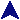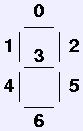CSc 232 Assignment 3CSc 232 Ruby Assignments### Due: April 6

Create two classes using the posted circuit simulation examples.The first one simulates a the common seven-segment display that looks like a squared-off 8. The device take seven input connections, each controlling one segment. Each segment is turned on or off by the value of one input bit, numbered from the right, as shown in figure. You can simulate this in text by using the underscore character for the horizontal lines, and the vertical bar character for the verticals. For instance, the simple test program:

require "segdisp"

sb = SwitchBank.new
ss = SevenSeg.new

7.times { sb.join(ss) }

sb.value = 0x57
sb.value = 0x6d
Produces the output
_
| |
|_
_
_|
_|
The first form is produced by hex 57, which, in binary is 101 0111. Compare this to the diagram to see that the segments where one bits appear (0, 1, 2, 4 and 6) are displayed, while the others are not. Likewise, the 3 form is produced by hex 6d, which is 110 1101 in binary. You should implement this in Ruby using the class name `SevenSeg`. Derive your class from `NumberOut` or one if its descendents.

The second thing you need to create is a decoder so that a four-bit number may be properly shown on the seven-segment display. This unit has four inputs for the number to display, and seven outputs for the seven display inputs. You should create it using gates, and construct class Decoder derived from Blueprint. Here's a small driver:

require "segdisp"
require "decode"

bp = Decoder.new

sb = SwitchBank.new
dc = bp.another
ss = SevenSeg.new

NumberOut.shush
7.times { dc.join(ss) }
4.times { sb.join(dc) }
NumberOut.shush(false)

sb.value = 4
sb.value = 9
sb.value = 12
And its output

|_|
|
_
|_|
_|
_
|
|_
The number goes into the decoder, and it produces the right signals to display it and the seven-segment device. For inputs over nine, the decoder produces a representation of the hex digit. Here are all the forms:
_       _   _       _   _   _   _   _   _       _       _   _
| |   |  _|  _| |_| |_  |_    | |_| |_| |_| |_  |    _| |_  |_
|_|   | |_   _|   |  _| |_|   | |_|  _| | | |_| |_  |_| |_  |
To get these, you must decide, for each four-bit input, whether each sigment should be lighted or not. For each segment (each output bit), you produce a boolean expression of the input bits which determines the if the segment is on (the value of the output bit). You must then construct this expression using the gate classes from the simulation. I've given some boolean expressions below which you may use; you'll still have to build the simulated gate circuits.

### Segment Expressions

Below are expressions for each of the seven segment output values in terms of the four input values. Input bits are numbered from the right, as usual. Setting the terms adjacent means "and," the plus sign means "or" and ¬ means "not".
s0 = i1i3) + i1 i2 + (¬i0) i1 + (¬i0) i3 + (¬i0) (¬i2) + i0 i2i3) + (¬i1) (¬i2) i3
s1 = i1 i3 + (¬i2) i3 + (¬i0) i2 + (¬i0) (¬i1) (¬i2) + (¬i1) i2i3)
s2 = (¬i2) (¬i3) + (¬i1) (¬i2) + (¬i0) (¬i1) (¬i3) + i0 i1i3) + i0i1) i3 + (¬i0) (¬i2)
s3 = (¬i0) i1 + (¬i2) i3 + i1 i3 + i1i2) + (¬i1) i2i3) + i0i1) i2
s4 = i2 i3 + i1 i3 + (¬i0) i1 + (¬i0) (¬i2)
s5 = i2i3) + (¬i2) i3 + i0i1) + (¬i1) (¬i3) + i0i3)
s6 = (¬i1) i3 + (¬i0) (¬i2) (¬i3) + i0 i1i2) + (¬i0) i1 i2 + i0i1) i2

### Submission

When your program works, is well-commented, and properly indented, submit it here.